How Cheenta works to ensure student success?
Explore the Back-Story

# Test of Mathematics Solution Subjective 36 - Invariance PrincipleTest of Mathematics Solution Subjective 36 (from ISI Entrance). The book, Test of Mathematics at 10+2 Level is Published by East West Press. This problem book is indispensable for the preparation of I.S.I. B.Stat and B.Math Entrance.

## Problem

Let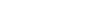be n numbers such that each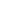is either 1 or -1. If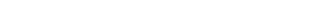then prove that 4 divides n.

## Solution

Let us denote each product of four numbers as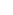. For example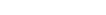. Note that the last one begins with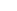indicating there are n's.

We check the equation modulo 4 (that is in each step we change 'something' in the equation and check what happens to the remainder of the sum when divided by 4).In the first step we note that equation is 0 mod 4 (since 0 when divided by 4 gives 0 as the remainder).

Next we convert one of the a's which is -1 into +1. Note that eachappears in exactly 4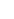. Hence converting the onefrom -1 to +1 will alter the values of 4Thus we may have five cases:

• All the four b's were -1. Hence after conversion all of their values will change into +1. Therefore the value of the total sum will increase by +8 (as previously the four b's added up to -4 and now they add up to +4). Thus no change of the sum modulo 4.
• Exactly three b's were -1 and the remaining was +1. Hence after conversion three of their values will change into +1 and the remaining one changes from +1 to -1. Therefore the value of the total sum will increase by +4 (as previously the four b's added up to -2 ((-1) + (-1) + (-1) + (+1)) and now they add up to +2). Thus no change of the whole sum modulo four.
• Exactly two b's were -1 and the remaining two were +1. Hence after conversion two of their values will change into +1 and the remaining two will change from +1 to -1. Therefore the value of the total sum will increase by 0 (as previously the four b's added up to 0 ((-1) + (-1) + (+1) + (+1)) and now they add up to 0). Thus no change of the whole sum modulo four.
• Exactly one b was -1 and the remaining were +1. Hence after conversion three of their values will change into -1 and the remaining one changes from -1 to +1. Therefore the value of the total sum will decrease by 4 (as previously the four b's added up to 2 ((+1) + (+1) + (+1) + (-1)) and now they add up to -2). Thus no change of the whole sum modulo four.
• All the four b's were +1. Hence after conversion all of their values will change into -1. Therefore the value of the total sum will decrease by 8 (as previously the four b's added up to +4 and now they add up to -4). Thus no change of the sum modulo 4.

Thus we see that changing one-1 to +1 does not change the value of the total sum modulo 4. Similarly we change all negative one's into positive one's (and in each step the sum remains invariant in modulo 4) to get n. Thus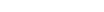. This implies 4 divides n.

## Key Idea

Modular arithmetic, invariance principle.Test of Mathematics Solution Subjective 36 (from ISI Entrance). The book, Test of Mathematics at 10+2 Level is Published by East West Press. This problem book is indispensable for the preparation of I.S.I. B.Stat and B.Math Entrance.

## Problem

Letbe n numbers such that eachis either 1 or -1. Ifthen prove that 4 divides n.

## Solution

Let us denote each product of four numbers as. For example. Note that the last one begins withindicating there are n's.

We check the equation modulo 4 (that is in each step we change 'something' in the equation and check what happens to the remainder of the sum when divided by 4).In the first step we note that equation is 0 mod 4 (since 0 when divided by 4 gives 0 as the remainder).

Next we convert one of the a's which is -1 into +1. Note that eachappears in exactly 4. Hence converting the onefrom -1 to +1 will alter the values of 4Thus we may have five cases:

• All the four b's were -1. Hence after conversion all of their values will change into +1. Therefore the value of the total sum will increase by +8 (as previously the four b's added up to -4 and now they add up to +4). Thus no change of the sum modulo 4.
• Exactly three b's were -1 and the remaining was +1. Hence after conversion three of their values will change into +1 and the remaining one changes from +1 to -1. Therefore the value of the total sum will increase by +4 (as previously the four b's added up to -2 ((-1) + (-1) + (-1) + (+1)) and now they add up to +2). Thus no change of the whole sum modulo four.
• Exactly two b's were -1 and the remaining two were +1. Hence after conversion two of their values will change into +1 and the remaining two will change from +1 to -1. Therefore the value of the total sum will increase by 0 (as previously the four b's added up to 0 ((-1) + (-1) + (+1) + (+1)) and now they add up to 0). Thus no change of the whole sum modulo four.
• Exactly one b was -1 and the remaining were +1. Hence after conversion three of their values will change into -1 and the remaining one changes from -1 to +1. Therefore the value of the total sum will decrease by 4 (as previously the four b's added up to 2 ((+1) + (+1) + (+1) + (-1)) and now they add up to -2). Thus no change of the whole sum modulo four.
• All the four b's were +1. Hence after conversion all of their values will change into -1. Therefore the value of the total sum will decrease by 8 (as previously the four b's added up to +4 and now they add up to -4). Thus no change of the sum modulo 4.

Thus we see that changing one-1 to +1 does not change the value of the total sum modulo 4. Similarly we change all negative one's into positive one's (and in each step the sum remains invariant in modulo 4) to get n. Thus. This implies 4 divides n.

## Key Idea

Modular arithmetic, invariance principle.

### Knowledge Partner